# CH 105 - Chemistry and Society

### Chemical Reactions

02/13/2008

Reaction Types

Think of the incredible number of chemical reactions going on around you and in you all the time. Each of your cells as you reading this is orchestrating thousands of different chemical reactions simultaneously. Since the advent of alchemy people have been making new types of compounds that never existed before. Luckily, the myriad of different chemical reactions can be reduced to a few types. All of these will be observed in Lab Experiment 3 in which pure copper foil will be converted through a series of chemical reactions to other substances and ultimately back to copper.  Several schemes have been developed to categorize chemical reactions.  The one we will use most often is charge transfer:    These reactions involve making and breaking bonds and the transfer of charge between substances.  If a proton is transferred from one substance to another, the reaction is called an acid/base reaction.  An electron transfer occurs in an oxidation/reduction reactions.  Finally soluble ions of salts can be "transferred" towards each other in solution and form an insoluble solid.  This reaction is called a precipitation reaction.

For describing and writing chemical reactions, we need to know the names and formulas of the compounds we will discuss.  In addiiton, we need to know how to write and balance chemical equations.  Naming ionic and molecular compounds is described in the figures below.  These diagrams are adapted from Chemistry by Atkins.   We will discuss balancing chemical equations in class.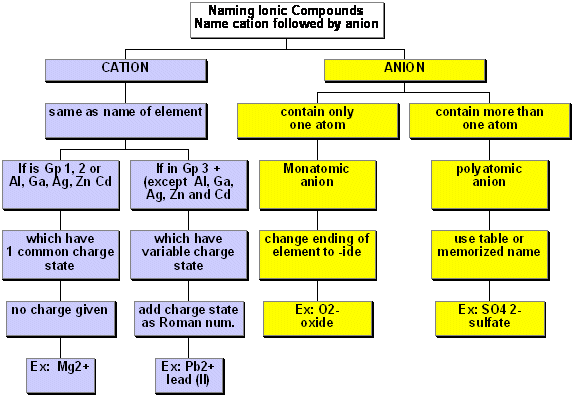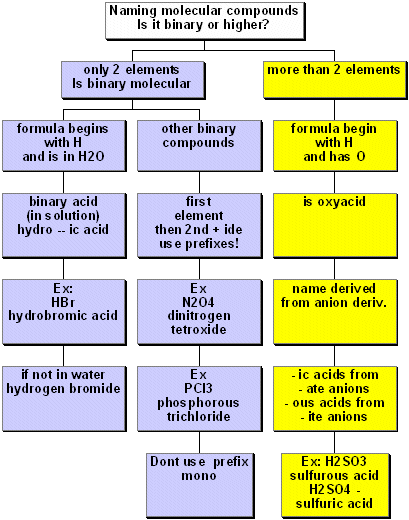Many reactions that we are interested in occur in solution, usually in water.  Some substances are soluble in water, others aren't.  We need to know what is actually in solution to figure out what type of reaction might occur.   A demo in class will attempt to show what is actually in solution.  A figure summarizing that is shown below. Some of the terms in the figure will be explained below.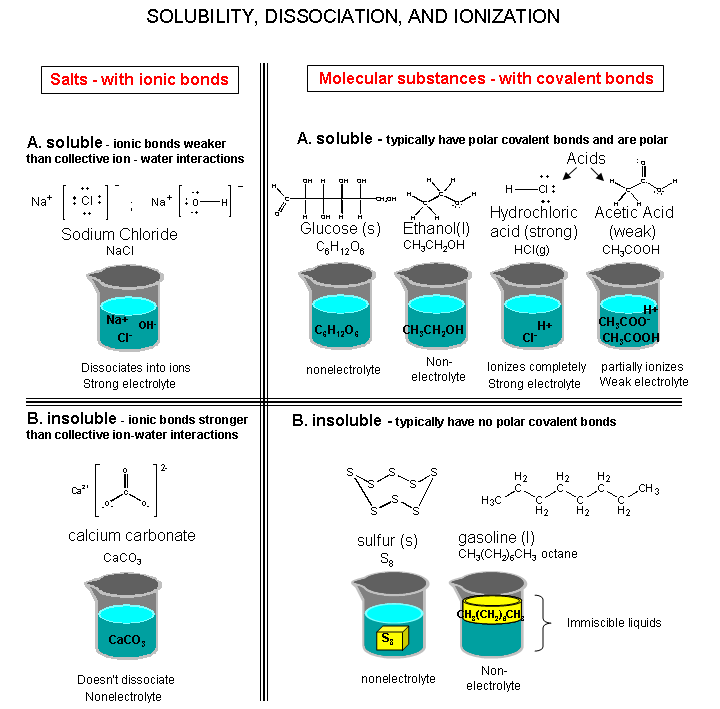Precipitation Reactions

Precipitation reactions occur when different aqueous solutions (usually of salts) are mixed, and a solid substance results. Remember solutions are homogeneous mixtures in which each part of the solution has the same composition. A solution can be colored (such as a blue copper sulfate solution) but it is always clear. Clear means that the solution is transparent, not cloudy, turbid, translucent, or opaque.

Most of the precipitation reactions that we will deal with involve aqueous salt solutions. Remember salts are compounds which consist of metal cations like Na+, Ca2+, Cu2+ (or the one nonmetal molecular ion that we have discussed, ammonium - NH4+) ionically bonded to nonmetal anions such as Cl-, (or molecular anions such as hydroxide - OH-, sulfate - SO42-, phosphate - PO43-, nitrate - NO3-, and carbonate - CO32-), dissolved in water. Salts can be divided into two types: those soluble in water, and those insoluble in water. You should know some simple solubility rules which will allow you to know which salts are soluble in water.

• nitrate NO3- salts are soluble
• salts containing Group 1 metals (Li, Na, K,  1+ charge) and NH4+ are soluble
• most Cl-, Br-, and I -salts are soluble, with the notable exceptions of salts that contain silver, Ag+ and lead, Pb2+
• most sulfate SO42- are soluble with the exceptions of salts containing barium, Ba2+, Pb2+ and Ca2+
• most hydroxides OH- are just slightly soluble, with the exceptions of the very soluble NaOH and KOH
• most phosphates PO43- and carbonates - CO32- are only slightly soluble

When salts dissolve in water, they seem to "melt away". What happened to the ions. An import clue comes from the fact that the resulting solution conducts electricity while pure water doesn't. We demonstrated this but putting a light bulb connected to two electrodes into water or a sodium chloride solution and connected it to an electrical outlet, as shown below.

Before we can understand this, we need to have a quick review of electricity. As a water current is a flow of water, a current of electricity can be viewed as a flow of electrons. Consider our hypothetical copper wire. Copper is a metallic element, but does it exists as copper atoms in the wire? This can't be since atoms of any element except the Group VIII Nobel gas elements are reactive. Something must be holding the copper particles together and cause the wire to be a good conductor of electricity (i.e. it can carry a current of electrons). In fact, the copper particles don't exist as atom but rather as positive ions which have given up an outer shell electron. These copper ions surrounded by a sea of mobile electrons account for the stability of the solid copper and the fact that it can conduct electricity - which is a flow of charged particles - in this case of electrons.

Why does a solution of NaCl conduct a current? Somehow when NaCl dissolves, it must formed individually charged particles. We know the NaCl consists of an array of Na+ and Cl- ions. If when NaCl dissolves, it separates into individual molecules of neutral NaCl, then the solution would not contain particles with an overall net charge. This suggests that the individual ions which comprise a "molecule" of the NaCl separate from each other -i.e. they dissociate - in water.  The O (slightly negative) on water interacts with the metal ion (positively charged cation), while the H (slightly positive) on water interacts with the nonmetal ion (negatively charged anion), separating the two ions. Charge particles are required for a flow of electric current. If the molecules of salt didn't separate into ions, the smallest particles in solution would be neutral salt molecules. NaCl and other soluble salts are strong electrolytes.

Precipitation reactions occur when different salt solutions are mixed, which result in the formation of an insoluble salt, or precipitate. When precipitation occurs, the cation of one of the soluble salts interacts with the anion of the other soluble salt to form an insoluble salt. The other ions which remain soluble are called spectator ions.. Consider the following example when aqueous solutions of NaCl and AgNO3 are added to each other. A white cloudy precipitate forms. What is the likely product that formed a precipitate? Consider all the ions in solution. If the positive ions approached each other, they would repel, so we can't form compounds like NaAg. The same is true of the negative ions. Ions of opposite charge can attract each other so we could reform NaCl and AgNO3. However, these are soluble salts and would immediately dissociate again into individual ions. The other possibilities are AgCl and NaNO3. The later would likewise dissociate since we know that Na and NO3 are soluble. The only alternative is AgCl. We can write a series of chemical equations to explain these interactions:

molecular equation: write down the species as molecules as below-

NaCl (aq) + AgNO3 (aq) --> AgCl (s) + NaNO3 (aq) where the (aq) for aqueous implies that the salt is in solution as is soluble.

ionic equation: write down the species as they actually occur in the solution:

Na+(aq) + Cl-(aq) + Ag+(aq) + NO3-(aq) --> AgCl(s) + Na+(aq) + NO3-(aq)

net ionic equation: remove identical ions that appear on both sides fo the ionic equation that act as "spectators" in the reaction. They are called spectator ions.

Ag+(aq) + Cl-(aq) --> AgCl(s)

Precipitation reactions form bone, teeth, kidney stones and gall stones.  We will not spend anymore time on these reactions.

Breaking Bonds:  Charge transfer reactions - Acid/Base and Oxidation/Reduction (Redox) Reactions

Chemical reactions involve making and breaking bonds. So far we have considered making and breaking ionic bonds between ions in aqueous salt solutions. Now we want to discuss making and breaking covalent bonds. When we break a covalent bonds, there are three ways we can separate the two atoms involved in the bond. Consider for example a C-X bond. If when the bond is broken, the electrons are left with C, C takes on a negative charge and is called a carbanion. If the electrons leave with X, then C is left with a positive charge and is a carbocation. If one electron stays with each atoms, the C has no charge, but does not have an octet. The carbon is called a free radical.

When the two electrons in a bond between X and H remain with X, a proton (H without its electron) is cleaved from the molecule.  An isolated proton is very reactive and will bind to another molecule which can accept it.  A reaction in which a proton is donated to another molecule is called an acid/base reaction.  If electrons are transferred from one substance to another, the reaction is called a oxidation/reduction (redox reaction).  We will first consider acid/base reactions.

Acid/Base Reactions

Lewis Structures:  Chemical Reactivity

From Lewis structures, and an understanding of formal and partial charges, you can predict how certain molecules react chemically with other molecules - i.e. how bonds in a molecule and broken and made.  Remember Lewis structures show only outer shell electrons, the one which are most likely shared or transferred in chemical reactions.  Molecules with atoms that have slight or full negative charges can "attack" molecules with atoms have a slight or full positive charge in the process of bond making and breaking.  The best example to illustrate this principle is for acid/base reactions.

Acid/base reactions are extremely important in biology and chemistry.   The reactions can be visualized as an attach by the slightly negative of the oxygen on water with the slightly positive hydrogen on an acid, as shown below.  An acid is defined as a proton donor, and it reacts to donate the proton to an electronegative, slightly negative atom on the proton acceptor, defined as an base.

 New 2/13/08  A note on nomenclatureIn the links above, you will see reaction mechanisms used curved arrows for bond making and breaking.  The arrows always start with a nonbonded pair of electrons on the base which is attracted to a δH+ on the acid or proton donor.  Hence the attack starts with the base.  That seems to be the opposite to the notion that an acid "actively" donates a proton.  From the perspective of the H+ on the acid, the H+ moves (is donated) to the base.  From the perspective of the lone pair on the O of the base, the base takes (accepts) the H+.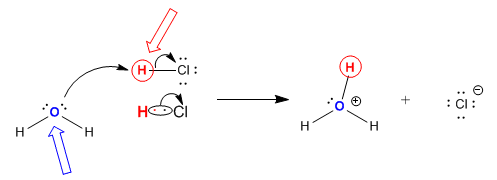To simplify this description, let's come up with a different description of an acid and base that indicate action initiated by the base (see mechanism above using curved arrows). ACID: molecule from which a proton (H+) is pulled by accepting an electron pair to a Hδ+ BASE: molecule which attacks and bonds to a proton (H+) by donating an elecron pair to a Hδ+

First, let's backtrack and consider what types of substances, when dissolved in water, allow for the conductance of an electric current. Remember, for the light to light, ions must be present in solution. Previously, we saw that an aqueous NaCl solution conducted electricity. Obviously the ionic bonds holding Na and Cl must be broken when solid NaCl dissolves in water which results in separate Na+ and Cl- ions. What about MgCl2? Of course it must since it is a salt, held together with ionic bonds, that dissolves in water and forms ions. What about AgNO3? Likewise this should as well since it is also a salt of the metal Ag and the polyatomic ion NO3- which separate into individual Ag+ and NO3-ions. (Remember the N and O atoms in this polyatomic or molecular ion, which are held together by covalent bonds, stay together).

Now let's consider compounds not held together by ionic bonds. Consider for example, methanol, [CH3OH(l)] and glucose [C6H12O6(s)]. There structures are shown below, along with silver nitrate. Remember silver nitrate is an ionic compound whose negative anion is a molecular ion, NO3-: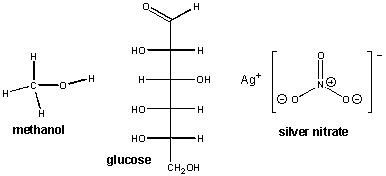When these substances are dissolved in water, no light is  observed when electrodes are placed in the water.  This implies that are no ions in solution. From these examples, we can tentatively conclude that covalently bonded species don't dissolve in water to form ions. This makes sense in that pure H2O itself, a molecule held together by polar covalent bonds, did not conduct electricity in our experiment.

But if  we did the same experiment with HCl(aq) added to water,  a bright light is  observed.

The only way we could get a light in this solution is if charged ions were present. Yet HCl is a neutral molecule. How could such charged ions be produced. The best possibility is that the H-Cl bond was broken and the electrons went to Cl making it Cl- with 8 outer shell electrons. We saw a similar situation when salts dissolved and the ions that comprised the salt dissociated (or separated from each other) to form individual ions.  The case of HCl is different.  It is a covalently bonded molecule - i.e. it is not made up of ions. However, when the HCl bond breaks, ions are formed.  We call this process ionization (compared to dissociation of ionically bonded salts).  The H would be left with no electrons and becomes H+ which is simple an isolated proton.  Classes of molecules like HCl that are bonded covalently yet seem to give up a proton in water when dissolved are called acids. Earlier definitions of acids were that they formed H+ in solutions.

The bond between H and Cl is not just severed by itself. Another species in solution - in this case water - attacks the HCl and pulls off the proton. The HCl donates a proton, our definition of an acid, and the water accepts a proton. A proton acceptor is called a base.

The acid/base reaction discussed above can be summarized by the equation:

HCl(aq) + H2O(l) ---> H3O+(aq) + Cl-(aq).

Again, H+ ions aren't just formed in solution. Rather an agent, water in this case, pulls the H ion off to form H3O+ . Just as in redox reactions, electrons are not simply lost when a substance gets oxidized, but rather are "pulled off" by a oxidizing agent which gets reduced in the process. The agent that pulls off the proton (H+) from HCl is water. The agent that donates protons (HCl) is an acid, the agent that accepts protons (H2O) is a base. Bases must have lone pair electrons so they can accept a proton.

In the reaction: HCl(aq) + H2O(l) ---> H3O+(aq) + Cl-(aq)

• HCl(aq) is the acid - a proton donor
• H2O(l) is the base - the proton acceptor

If you look at the products, you could imagine they could also react in a reverse of the original reaction to produce the original reactants.

H3O+ + Cl- ---> HCl(aq) + H2O(l)

In this reaction:

• H3O+ is the acid - a proton donor
• Cl- is the base - the proton acceptor

Why doesn't this reverse reaction also occur?  It actually does to an extremely small extent we can barely measure any unionized HCl. You could actually envision the original reaction as reversible:

HCl(aq)A + H2O(l)B <===> H3O+(aq)A + Cl-(aq)B

where A indicates which reactants/products are potential acids in the forward and reverse reactions, and B indicates potential bases. Which way does this reaction go?   The reaction goes in the direction from the stronger acid and base to the weaker acid and base. In the above example, HCl(aq) is the stronger A and H2O(l) the stronger B.   Strong acids such as nitric acid, sulfuric acid, hydrochloric acid are essentially completely ionized - they are strong electrolytes.

The simplest kind of acid base reaction that we experience often in the lab is that of a strong acid with a strong base. For us, the strong bases are those that contain OH- ions. Hence

HCl(aq)A + NaOH(aq)B <===> H2O (l)A + NaCl(aq)B - molecular eq.

Note: a strong acid reacts with a strong base to form a salt and water - the acid and base are neutralized.

Other acids are not strong, but weak.  They hold onto their proton more tightly.  An example is acetic acid.

CH3CO2H  +  H2O  <==>  H3O+  +  CH3CO2-

Acetic acid is a weak acid.  Only one molecule of acetic acid out of 10,000 in solution gives up its proton  to water molecules in solution.  If you hooked up the electrode and light bulb with a dilute acetic acid solutions, you would get at best a faint glow.  Acetic acid in water is a weak electrolyte.

You could coax every acetic acid molecule in solution to all give up proton.  To do this, you would need to add a stronger base than water, for example NaOH. Why would OH- be a stronger base (i.e. more likely to accept a  proton (H+) than is water. ?

Water can even act as an acid with another molecule of water, which acts as a base, to form H3O+ and OH-, a strong acid and base respectively.

H2O  +  H2O  <==>  H3O+  +  OH-

Water is even a weaker acid than acetic acid.   Only about 1 in a billion  water molecules reacts with another water molecule to form H.

 New 2/10/08:  pH You've heard of pH in earlier chemistry and biology classes.  It's a measurement of the concentration of H3O+, [H3O+], in solution.  Obviously if you add HCl to water, this strong acid essentially completely reactions with water to form an equivalent amount of H3O+ and Cl-.  pH is defined as follows:  pH = -log  [H3O+].  In chemistry, we usually measure the concentration of a substance in mass of solute/ volume solution.  The most useful of mass is not grams but moles (which we will discuss later in the stoichiometry section), and volume is in liters (L).  The concentration units are then moles solute/L solution.  A solution of 1 mole of a solute in one liter of solution has a concentration of 1 Molar (M).    In pure water, the amount of  H3O+  and  OH- produced from the reaction: H2O  +  H2O  <==>  H3O+  +  OH- are equal and equal to 1 x 10-7 M.  Hence the pH of pure water is: pH = -log  [H3O+] =  -log  [10-7] = 7. Remember this easy trick for simple logs: log 100 = log 102 = 2;  log 10 = log 101 = 1; log 0.1 = log 10-1 = -1; log 0.01 = log 10-2 = -2

To emphasize once more, acids vary in strength.  They react in water to form H3O+ (aq).  Strong acids completely give up protons to water, weak acid  give up protons to water poorly.  If you had a billion (1,000,000,000) molecules of the following acids in water, how many of them would react to donate a proton to water which acts as a base?  Check out the table below:

 Acid Strength How many donate their proton? HCl strong ≈ 1,000,000,0000 CH3CO2H weak ≈ 100,000 H2O even weaker ≈ 1
 New 2/10/08:  The pH of pure water is 7.  The pH of a 0.1 M acetic acid solution is about 5 since the actual [H3O+] is about 0.00001 or 10-5 M.  The pH of a 0.1 M HCl solution = 1, since all the HCl has reacted to produce 0.1 M  [H3O+].  If you raised the pH of the 0.1 M acetic acid solution by adding a stronger base (like NaOH) than water , the pH would increase.  At pH 7.0, most of the acetic acid molecules have donated their protons to OH- to form water. (not H3O+).

There are also examples of weak bases (proton acceptors). For example, neutral NH3, ammonia, can accept a proton from an acid to form NH4+ much like neutral water, H2O, an accept a proton to form  H3O+ and OH-.     or

NH3 + H2O  <==>  NH4+ + OH-

Acids similar to acetic acid and bases similar to ammonia are found in large biological molecules called proteins.  If their acetic acid-like and ammonia-like groups react with water, they will form negative and positively charged groups, respectively.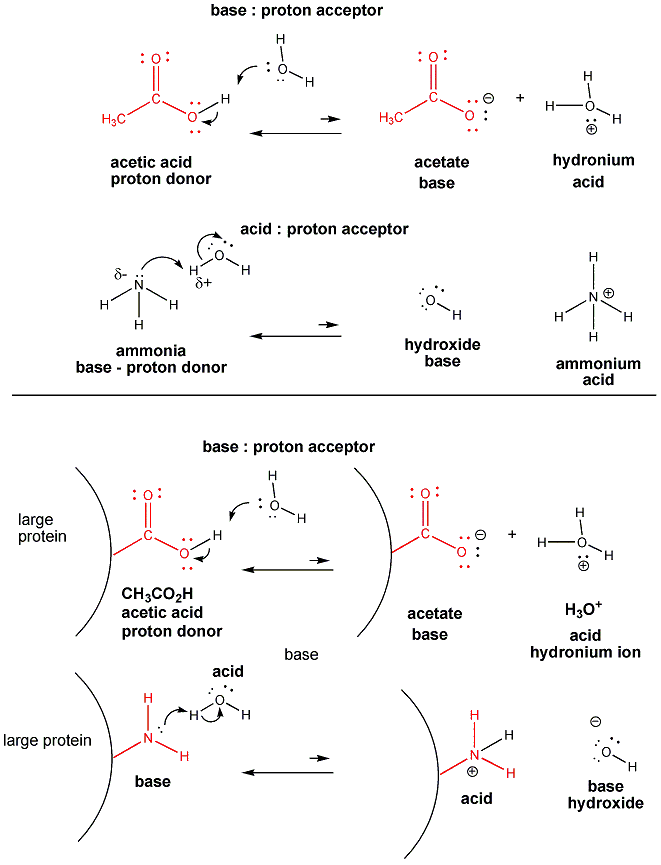Acid base reactions are very importantly biologically since the pH of the blood and cells, a measure of the acidity of the blood, must be maintained at very precise levels.  They are very important for us when we realize the biological molecules like DNA, RNA, proteins, and fats, can have charges since they often contain acetic acid-like and ammonia-like groups which can react as acids and bases, respectively, with water to form negatively and positively charged ions attached to the biological molecule.

Quiz: Acid/Base

Summary:  What's in the Beaker - Solubility, Dissociation, and Ionization

Oxidation/Reduction (Redox)  Reactions

We have just discussed acid/base reactions, which involve proton transfer. Now we will discuss another kind of charge transfer, electron  transfer or oxidation/reduction  reactions. In oxidation/reduction reactions, there is a transfer of charge - an electron - from one species to another. Oxidation is the loss of electrons and reduction is a gain in electrons.  Use these acronyms to help you remember:  Leo Ger - Loss of electrons oxidation; Gain of electrons reduction or Oil Rig - Oxidation involves loss, Reduction involves gain - of electrons.) These reactions always occur in pair. That is, an oxidation is always coupled to a reduction. When something gets oxidized, another agent gains those electrons, acting as the oxidizing agent, and gets reduced in the process. When a substance gets reduced, it gains electrons from something that gave them up, the reducing agent, which in the process gets oxidized.  It's just like an acid base reaction.  An acid reacts with a base to form a new acid and base.

Reactions in which a pure metal reacts with a substance to form a salts are clearly oxidation reactions. Consider for example the reaction of sodium metal and chlorine gas.

2Na(s) + Cl2 -----> 2NaCl(s)

Na is a pure metal. (Although it really exists as sodium ions surrounded by a sea of electrons), consider it for our purposes to exist as elemental Na, which has a formal charge of 0. Likewise, Cl2 is a pure element. To determine the charge on each Cl atoms, we divide the two bonded electrons equally between the two Cl atoms, hence assigning 7 electrons to each Cl. Hence the formal charge on each Cl is 0.

In a similar fashion we can determine the oxidation number of an atom bonded to another atom. We can assign electrons to a bonded atoms, compare that number to the number in the outer shell of the unbonded atoms, and see if there is an excess or lack.  In these cases, the same number of electrons get assigned to each atoms as when we are calculating formal charge. Hence the oxidation numbers are equal to the formal charge in these examples. Clearly, Na went from an oxidation number and formal charge of 0 to 1+ and Cl from 0 to 1-. Therefore, Na was oxidized by the oxidizing agent Cl2, and Cl2 was reduced by the reducing agent Na.

Let's consider other similar redox reactions:

• 2Mg(s) + O2(g) ----> 2MgO(s)
• Fe(s) + O2(g) ---> Fe oxides (s)
• C(s) + O2(g) ----> CO2(g)

In the first two reactions, a pure metal (with formal charges and oxidation numbers of 0) lose electrons to form metal oxides, with positive metal ions. The oxygen goes form a formal charge and oxidation number of 0 to 2- and hence is reduced.

What about the last case? Each atom in both reactants and products has a formal charge of 0. This reaction, a combustion reactions with molecular oxygen, is also a redox reaction. Where are the electrons that are lost or gained?

This can be determined by assigning the electrons in the different molecules in a way slightly different than we did with formal charge. For shared (bonded) electrons, we give both electrons in the bond to the atom in the shared pair that has a higher electronegativity. Next we calculate the apparent charge on the atom by comparing the number of assigned electrons to the usual number of outer shell electrons in an atom (i.e. the group number). This apparent charge is called the oxidation number. When we use this method for the reaction of C to CO2, the C in carbon dioxide has an oxidation number of 4+ while the two oxygens have an oxidation number of 2- . Clearly, the C has "lost electrons" and has become oxidized by interacting with the oxidizing agent O2. as it went from C to CO2. If the atoms connected by a bond are identical, we split the electrons and assign one to each atom. In water, the O has an oxidation number of 2- while each H atom has an oxidation number of 1+. Notice that the sum of the oxidation numbers of the atoms in a species is equal to the net charge on that species.

What we have done is devise another way to count the electrons around an atom and the resulting charges on the atoms. See the animation below to review electron counting, and the 3 "types of charges" - partial charges, formal charges, and now oxidation numbers.

Consider an O-X bond, where X is any element other than F or O. Since O is the second most electronegative atom, the two electrons in the O-X bond will be assigned to O. In fact all the electrons around O (8) will be assigned to O, giving it always an oxidation number of 2-. This will be true for every molecule we will study this year except O2 and H2O2 (hydrogen peroxide). Now consider a C-H bond. Since C is nearer to F, O, and N than is H, we could expect C (en 2.5) to be more electronegative than H (en 2.1). Therefore, both electrons in the C-H bond are assigned to C, and H has an oxidation number of 1+. This will always be true for the molecules we study, except H-H. A quick summary of oxidation numbers shows that for the molecules we will study:

• O always has an oxidation # of 2- (except when it is bonded to itself or F)
• H always has an oxidation # of 1+ (except when it is bonded to itself)
• The sum of the oxidation numbers on a compound must equal the charge on the compound (just like the case of formal charges)

Notice in each of the reactions above, oxygen is an oxidizing agent. Also notice that in each of these reactions, a pure element is chemically changed into a compound with other elements. All pure, uncharged elements have formal charges and oxidation numbers of 0. When they appear as compounds in the products, they must have a different oxidation number. The disappearance or appearance of a pure element in a chemical reaction makes that reaction a redox reaction.

Now lets consider a more complicated case - the reaction of methane and oxygen to produce carbon dioxide and water:

CH4 + O2 -----> H2O + CO2.

Since H has an oxidation # of  1+, the oxidation # of C in CH4 is 4-, while in CO2 it is 4+. Clearly C has been oxidized by the oxidizing agent O2. O2 has been reduced to form both products.

Now consider a series of step-wise reactions of CH4 ultimately leading to CO2

.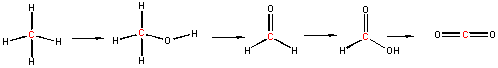You should be able to determine that the oxidation numbers for the central C in each molecule are 4-, 2-, 0, 2+, and 4+ as you proceed from left to right, and hence represent step-wise oxidations of the carbon. Stepwise oxidations of carbon by oxidizing agents different than O2 are the hallmark of biological oxidation reactions. Each step-wise step releases smaller amounts of energy, which can be handled by the body more readily that if it occurred in "one step", as indicated in the combustion of methane by O2 above.

You may have learned in a previous course that in oxidation reactions, there is an increase in the number of X-O bonds, where X is some atom. Alternatively, it also involves the decrease of X-H bonds. Reduction would be the opposite case - decreasing the number of X-O bonds and/or increasing the number of X-H bonds. This rule applies well to the above step-wise example.

Redox reactions are common in nature. Some common redox reactions are those that occur in batteries, when metals rust, when metals are plated from solutions, and of course combustion of organic molecules such as hydrocarbons (like methane and gasoline) and carbohydrates (like wood). A simple redox reaction that leads to the plating or deposition of a pure metal from a solution of that metal is shown below.

Cu(s) + 2Ag+(aq) ---> Cu2+(aq) + 2Ag(s)

In this reaction, pure silver metal - Ag(s) is plated on the surface of Cu(s) In this reaction:

• Cu is oxidized and is the reducing agent
• Ag+ is reduced and is the oxidizing agent

If you look at the products, you could imagine they could also react in a reverse of the original reaction to produce the original reactants.

Cu2+(aq) + 2Ag(s) ---> Cu(s) + 2Ag+(aq)

In this reaction, pure copper metal - Cu(s) would be plated on the surface of the Ag(s). In this reaction:

• Ag(s) is oxidized and is the reducing agent
• Cu2+ is reduced and is the oxidizing agent

Why doesn't this reverse reaction also occur? It actually does to a small extent. You could actually envision the original reaction as reversible:

Cu(s)RA + 2Ag+(aq)OA <===> Cu2+(aq)OA + 2Ag(s)RA

where RA indicates which reactants/products are potential reducing agents in the forward and reverse reactions, and OA indicates potential oxidizing agents. Which way does this reaction go? Suffice it to say, the reaction goes in the direction from the strongest oxidizing and reducing agents to the weakest. In the above example, Cu(s) is the stronger RA and Ag+ is the stronger OA.

Consider this reaction from Lab 3 when you add solid Zn to the blue copper sulfate solution to produce pure Cu(s):

Zn(s) + Cu2+(aq) ---> Zn2+(aq) + Cu(s)

This reaction can be thought of as 2 half-reactions which can be added together to get the top reaction:

Zn(s) + ---> Zn2+(aq) + 2e-

Cu2+(aq) + 2e- ---> Cu(s)

Image if you tried to separate the reactions into two beaker, one containing Zn(s) and the other Cu2+. Obviously the reactions could not occur. However, if we connected the two beakers with a wire (which would allow electrons to flow from Zn(s) to Cu2+(aq), then the reactions can occur. If we put a voltmeter or light bulb in between the two beakers, a voltage is recorded or the light bulb will light. We have made these redox reactions into a battery.

Redox Reactions - Voltage Cell and Battery

Quiz: Oxidation/Reduction

Summary of Reactions

Typical characteristics of these reactions are shown below:

• In precipitation reactions, an aqueous salt solution is mixed with another aqueous salt solution to form a solid precipitate, if one of the resulting salts is insoluble. If the new salts that can form are soluble, then no reaction occurs.
• In redox, reactions, a pure element is either consumed or formed in the chemical reaction. This would include all oxidation reactions involving O2.  A reaction which increases the number of bonds from an atom to oxygen represents an oxidation of that atom and a reduction of oxygen.  A decrease in the number of bonds from an atom to oxygen represents a reduction of the atom.  Both an oxidizing and reducing agent must be present.
• In acid/base reactions, an acid (such as HCl, HF, HNO3, H2SO4, CH3CO2H - acetic acid) reacts with a base (such as OH-, H2O, NH3). Look for both an acid and base as reactants.

Use those simple rules to classify simple reactions. Reactions involving acids can be tricky since their presence as a reactant does not imply that the reaction is an acid/base reaction, UNLESS A BASE IS PRESENT. This is also true of bases like OH- which can be a base or a precipitating agent. Tricky examples that we discussed include:

• H2SO4 (sulfuric acid) acts as an acid when a base (like H2O or OH- is present)
• H2SO4 (sulfuric acid) acts as a source of ions in precipitation reactions (i.e. it is a precipitating agent) when it interacts with a BaCl2 salt solution
• HNO3 (nitric acid) acts as an oxidizing agent when it interacts with Cu(s) as in Lab 3.
• NaOH acts as a source of OH- ions in precipitation reactions such as with Cu(NO3)2 in Lab 3.

Similarities in Reactions:

As there is a continuum in bond types, from ionic (no sharing of electrons to nonpolar covalent with a complete sharing of electrons), there is a continuum in

The truth is that even with insoluble salts, there are small concentrations of dissolved ions in solution - too small to allow our light to light. Likewise, H2O can react with itself in an acid/base reaction to produce low concentrations of ions (again not enough to light a light), as shown below

H2O + H2O <==> H3O+ + OH-

This reaction is an autoionization of water in which water acts both as an acid and base.

The Transformation of Copper

In lab 3 we transformed pure copper foil through a series of chemical intermediates back into pure copper again. Hopefully you were awed by the amazing chemical transformations that took place. Click on the underlined reactions below .to relive your laboratory experiences. Then take the Moodle quiz  to see how well you understood the reactions.

Hints when trying to predict products:

1. First look for obvious precipitation reactions - two aqueous salt solutions mixed together with one of the salts containing an ion that quite often is insoluble (such as Fe, Ba, Ag, Hg, Pb)
2. Then looks for other possible precipitation reactions in which one of the above ions is present in an aqueous salt but the other solution is an acid which might provide an anion (like sulfate or chloride) that could precipitate with the above ion.
3. Next identify easy acid/base reactions - in which you have an easily identifiable acid (nitric, sulfuric, hydrochloric) and a hydroxide salt as the base
4. Now look for easily identifiable redox reactions in which O2 is a reactant and probably an oxidizing agent and the other reagent is a hydrocarbon, a carbohydrate, a metal, etc. The hydrocarbon and carbohydrate will react to form carbon dioxide and water, and the metal will react to form oxides.
5. Finally look for other redox reactions, such as when you have a pure metal interacting with a ion of another metal or an acid which could dissolve it to form a salt of the metal.